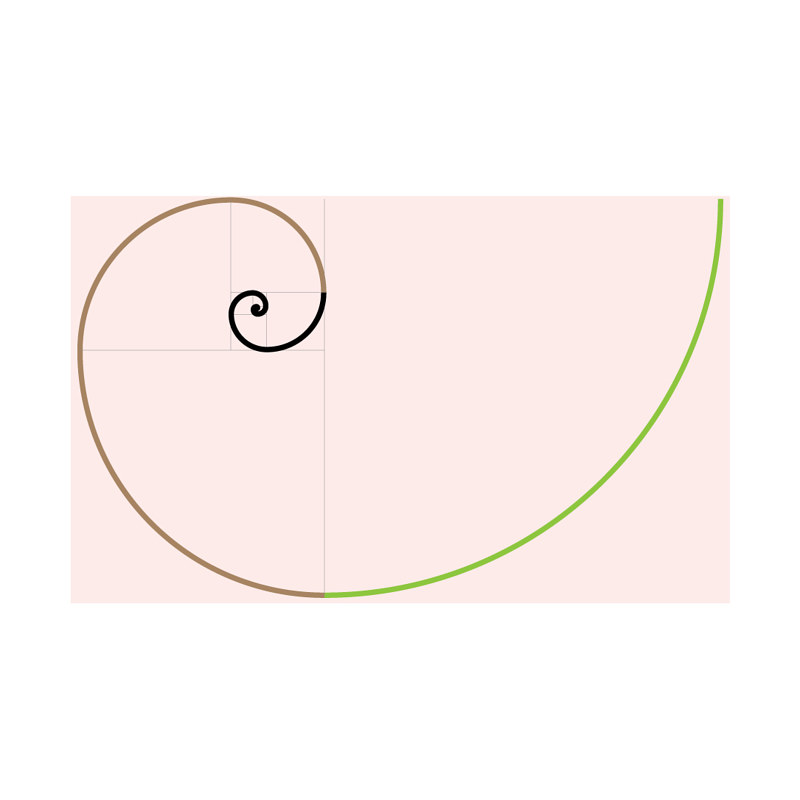Fibonacci Spiral

digital image, size variable 2020 FS0001

permission via en.wikipedia.org/wiki/Golden_spiral and en.wikipedia.org/wiki/Fibonacci_number
A Fibonacci spiral starts with a rectangle partitioned into 2 squares. In each step, a square the length of the rectangle's longest side is added to the rectangle. Since the ratio between consecutive Fibonacci numbers approaches the golden ratio as the Fibonacci numbers approach infinity, so too does this spiral get more similar to the previous approximation as more squares are added.